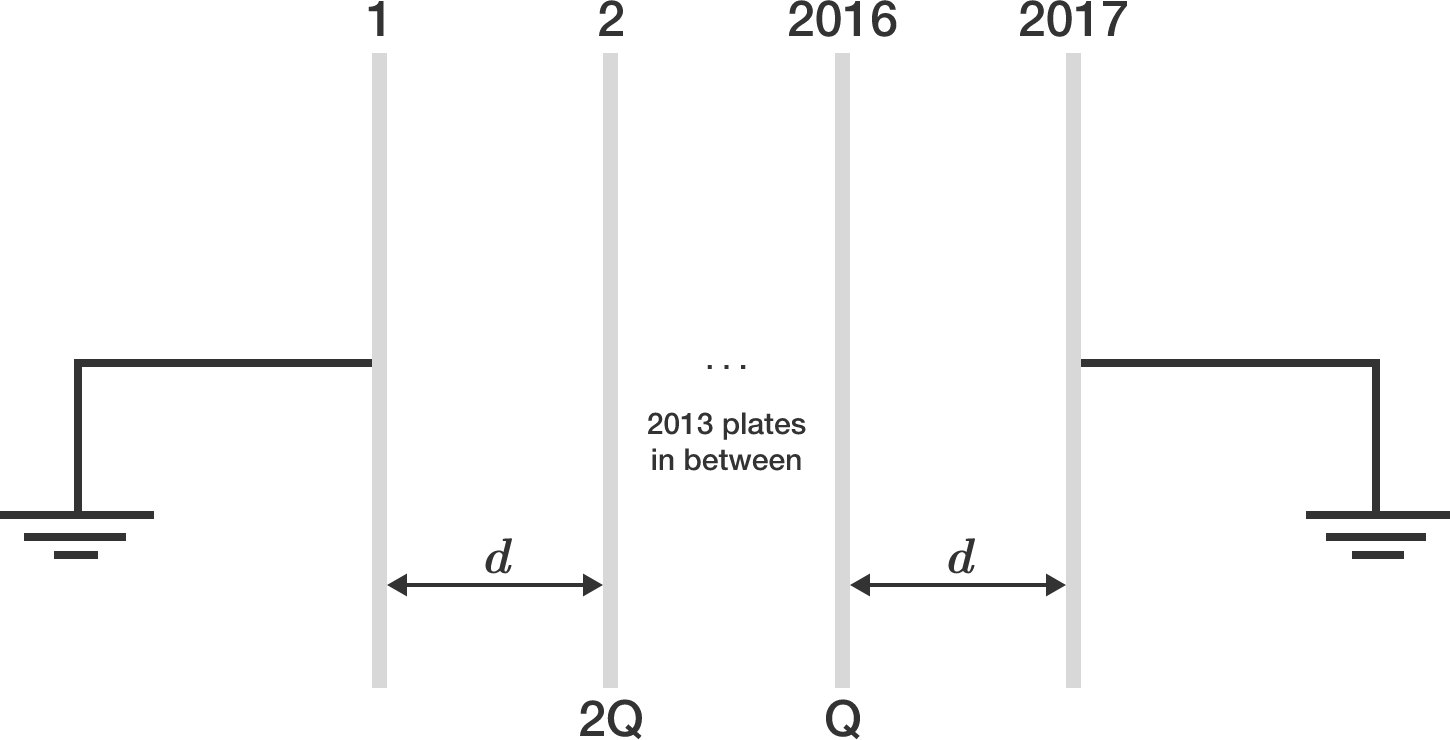# 2017 charged plates2017 identical metallic plates (initially uncharged), each having area of cross section $A$, are each separated by a distance of $d,$ as shown in the figure above.

Plates 2 and 2016 are given charges $2\mathbf Q$ and $\mathbf Q,$ respectively. Plates 1 and 2017 are both earthed via a very thin conducting wire.

If the potential difference between plates 1728 and 1729 is of the form $\dfrac{a Qd}{bA\epsilon_0}$, where $a$ and $b$ are coprime positive integers, then submit your answer as $a+b$.


Details and Assumptions:

• $\epsilon_0$ denotes the permittivity of free space.
• The plates are very large, and the phenomena of fringing of electric field lines are neglected.
• Because of the large size, the electric field due to any particular plate is considered as that of an infinitely large, thin conducting plate.
×